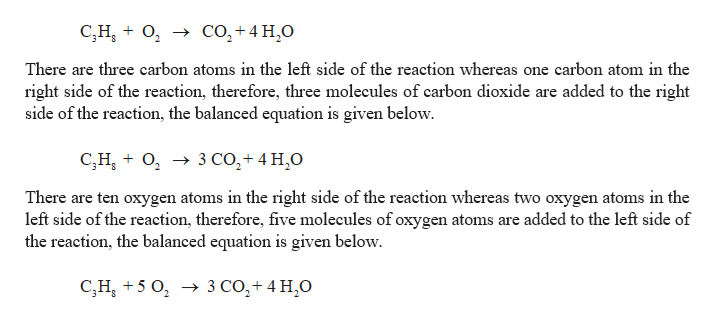# Consider the combustion reaction of propane, C3H8, with oxygen gas.a. Write the balanced chemical equation for this reaction.b. What mass of oxygen is needed to burn 100.0grams of propane?c. What is the theoretical yield of water when 100.0g of propane undergoes combustion?

Question
26 views

Consider the combustion reaction of propane, C3H8, with oxygen gas.
a. Write the balanced chemical equation for this reaction.
b. What mass of oxygen is needed to burn 100.0grams of propane?
c. What is the theoretical yield of water when 100.0g of propane undergoes combustion?

check_circle

Step 1

Combustion reaction:

Combustion is an exothermic reaction between a fuel (the reductant) and an oxidant. Oxidant is usually atmospheric oxygen.

Generally organic molecule undergoes combustion reaction which produces carbon dioxide and water molecule.

Balancing reaction:

Balanced reaction is a chemical reaction in which number of atoms for each element in the reaction and the total charge are same on both reactant side and the product side.

Steps in balancing the information

Step 1: Write the unbalanced equation

Step 2: Find the coefficient to balance the equation.

The coefficient should be reduced to the smallest whole number.

Propane undergoes combustion reaction with oxygen which produces carbon dioxide and water molecule.

Step 2

while balancing the equation, the subscripts cannot be altered but coefficients can be changed. There are eight hydrogen atoms in the left side of the reaction whereas two hydrogen atoms in the right side of the reaction, therefore, four molecules of water are added to the right side of the reaction, the balanced equation is given below.help_outlineImage TranscriptioncloseC,HO Co2+4 H0 There are three carbon atoms in the left side of the reaction whereas one carbon atom in the right side of the reaction, therefore, three molecules of carbon dioxide are added to the right side of the reaction, the balanced equation is given below. C,H0 3 CO,+4 H2O There are ten oxygen atoms in the right side of the reaction whereas two oxygen atoms in the left side of the reaction, therefore, five molecules of oxygen atoms are added to the left side of the reaction, the balanced equation is given below 3 CO2+ 4 H,0 C,H5 fullscreen
Step 3

(b) The mass of oxygen has to be calculated to burn 100.0 g of...

### Want to see the full answer?

See Solution

#### Want to see this answer and more?

Solutions are written by subject experts who are available 24/7. Questions are typically answered within 1 hour.*

See Solution
*Response times may vary by subject and question.
Tagged in

### Chemistry